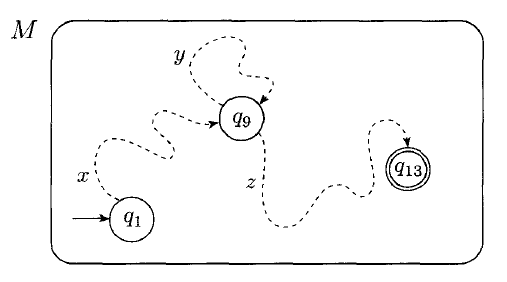# Non-Regular Languages

## Overview

We've seen several ways of representing regular languages:

• DFAs
• NFAs
• Regular Expressions

We can lots of interesting things with regular languages, but there are many languages that are not regular:

• The set of words with an equal number 0s and 1s?
• The set of words that are palindromes?
• The set of words whose length is a prime number?

These languages have no finite automata which recognizes them, and no regular expression which generates them.

Today we will look at how we can show that a language is not regular.

## The Pumping Lemma

The pumping lemma states that all regular languages have a certain property. If we can show that a language does not have that property, then it must not be regular.

The pumping lemma states:

If $A$ is a regular language, then there is a number $p$ where if $s$ is any string in $A$ of length at least $p$, then $s$ may be divided into three pieces $s = xyz$ satisfying the following:

1. $\forall i \geq 0, xy^iz \in A$
2. $|y| > 0$
3. $|xy| \leq p$

## Pumping Lemma Explanation

If $A$ is a regular language, then we know some DFA $M$ recognizes it. We can assign $p$ to be the number of states of $M$.

We then choose any string $s$ that is part of $A$ and has length at least $p$.

We then look at the states that $M$ is in as it computes $s$. It will visit exactly $n + 1$ states, when $n$ is the length of $s$:By the pigeonhole principle, we must visit some state twice!

The part of s that comes before the repeated state is $x$. The part between is $y$. The part after is $z$.This satisfies the three conditions:

1. No matter how many times we loop $y$, we will still have a string in $A$.
2. The string $y$ is not empty.
3. $xy$ cannot be greater than $p$, the number of states (else there would be another repetition).

The big idea here is that with a finite number of states, a DFA cannot produce strings of arbitrary length without at some point returning to the exact same state it had been at previously.

## Pumping Example

How can we demonstrate the pumping lemma for the language generated by $01^\ast0$?

## Proving Non-Regularity

The main use of the pumping lemma is to prove that languages are not regular. The basic idea is this:

Given a language $L$ we suspect to be non-regular,

1. Assume $L$ is regular, and that the pumping lemma holds.
2. Show that, for some choice of $p$ and $s$, the pumping lemma does not hold.
3. Conclude that the assumption of the regularity of $L$ was not true.

Note that, the pumping lemma holds for any choice of $p$ or $s$. A non-regular language may well have some strings in it that can be pumped!

## Example

Let $B = \{0^n1^n \ |\ n \geq 0\}$

1. Assume $B$ is regular.
2. Choose $s = 0^p1^p$. Because $s$ is a member of $B$, and $|s| \geq p$, the pumping lemma says we can split $s = xyz$ and that $\forall i \geq 0 xy^iz \in A$.

There are three cases for our choice of $y$:

1. $y$ consists entirely of zeroes. In this case, $xyyz$ will have more zeroes than ones and will no longer be a member of $B$.
2. Likewise, if $y$ contains only ones, looping it will produce strings with more ones than zeroes.
3. $y$ contains some zeroes and some ones. In this case $xyyz$ may have an equal number of zeroes and ones, but they will be out of order.

No matter the choice of $y$, we cannot pump $y$ without leading us to strings which are not part of our language.

Therefore the pumping lemma does not hold for $B$ and it must be non-regular.